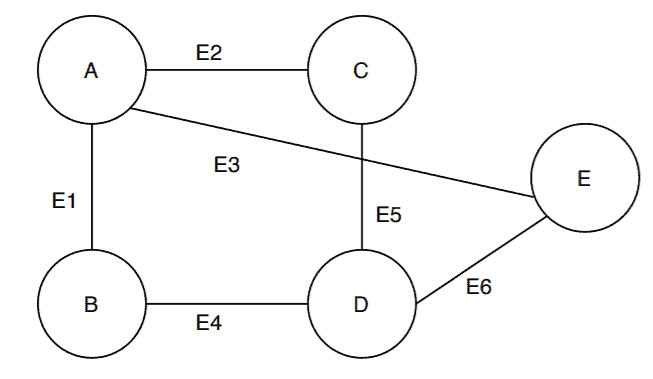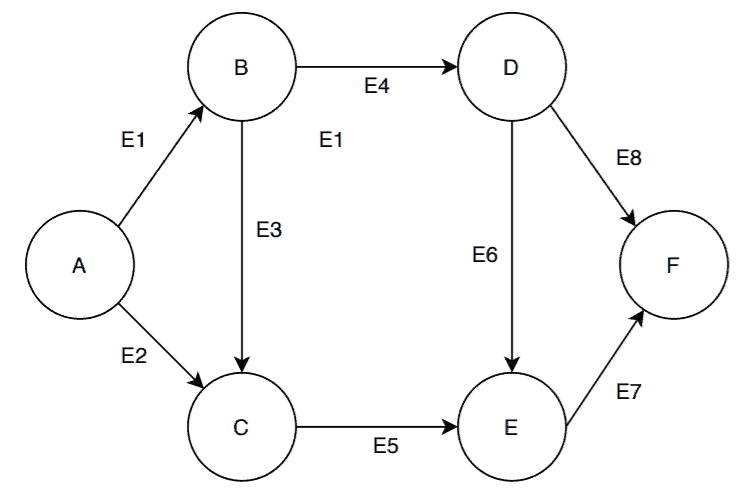## 1. Overview

In this tutorial, we’ll discuss what an incident edge is and how it can be found in directed and undirected graphs.

## 2. General Idea

In general, two edges are “incident” if they share a common vertex.

Not only edges, but vertices can also be incident with an edge. A vertex is incident with an edge if the vertex is one of the endpoints of that edge.

So, let’s assume we’ve got two edgesand. Further, assume that the edges have a common endpoint vertex. In this case, we can say the edgesandare incident.

Alternatively, if an edgeconnects two verticesandthen the edge is said to be incident on the vertexand.

Notice that in the previous example, the vertexis part of both the edgesand. In such cases, we can say that the vertexis incident on the edgesand.

## 3. An Undirected Graph Example

In this section, let’s try to find out some incident edges from our sample directed and undirected graphs.

We’re taking on an undirected graph first:This undirected graphhas a the vertex setand an edge set. From this graph, let’s find out some of the incident relationship among edges and vertices:

• The edges,,are incident edges as the vertexis one of their common endpoints
• Alternatively, the vertexis incident on the edges,,• The edgesandare incident edges as the vertexis one of their common endpoints
• Alternatively, the vertexis incident on the edgesandSimilarly, we can find other incident relationship between the edges and vertices in graph.

## 4. A Directed Graph Example

Let’s talk about a directed graph now:Now, we’ve taken a directed graphwith the vertex setand edge set. We’re aiming to define the incident relationship between the edges and vertices in:

• The edgesandare incident as they share the common vertex• The vertexis incident on the edgeand• The edgeis incident on the vertexfrom the vertex• Similarly, the edgeis incident on the vertexfrom the vertexBasically, the incident relationship between the vertices and edges in a directed as well as an undirected graph remains the same. The only difference is that in case of a directed graph, as the edges have directions so they are now incident on a vertex from another vertex.

## 5. Applications

In graph theory, if we want to calculate the degree of a vertex, we count the number of edges incident on the vertex. The total number of edges incident on a vertex is the degree of that particular vertex. So the incident edge concept is used to find out the degree of a vertex.

The incident edge concept is used in the edge coloring problem in graph theory. In edge coloring, all the edges need to fill with color such that no two incident edges have the same color.

Another use of the incident edge concept is in the edge cover problem. Edge cover consists of a set of edges and each vertex in the graph should incident on at least one of the edges from the edge cover set.

## 6. Conclusion

In this tutorial, we’ve discussed the incident edges and vertices in both directed and undirected graphs.

Furthermore, we’ve presented a couple of examples and mentioned some applications as well.